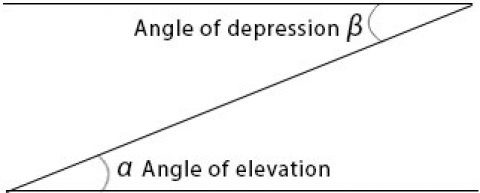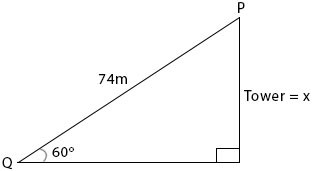### Trigonometry (II)

Mathematics SSS 1 Third Term

WEEK 6

Trigonometry (II)

Performance Objectives

Students should be able to;

1. Solve problems on the practical application of trigonometry ratios
2. Obtain sine and cosine of various angles
3. Identify the relationship between the trigonometric ratios and measured lengths

Application of Trigonometry

ContentAngle of elevation:

Example 1:

The angle of elevation of a point P on a tower from a point Q on the horizontal ground is 600. If /PQ/=74m, how high is P above the ground?Solution:

View More